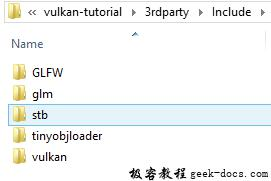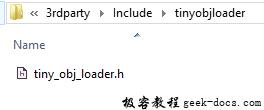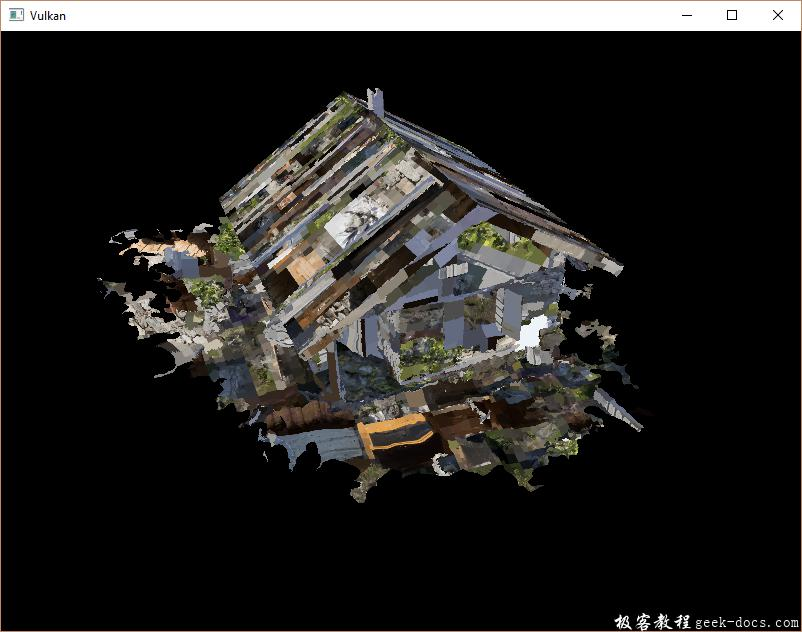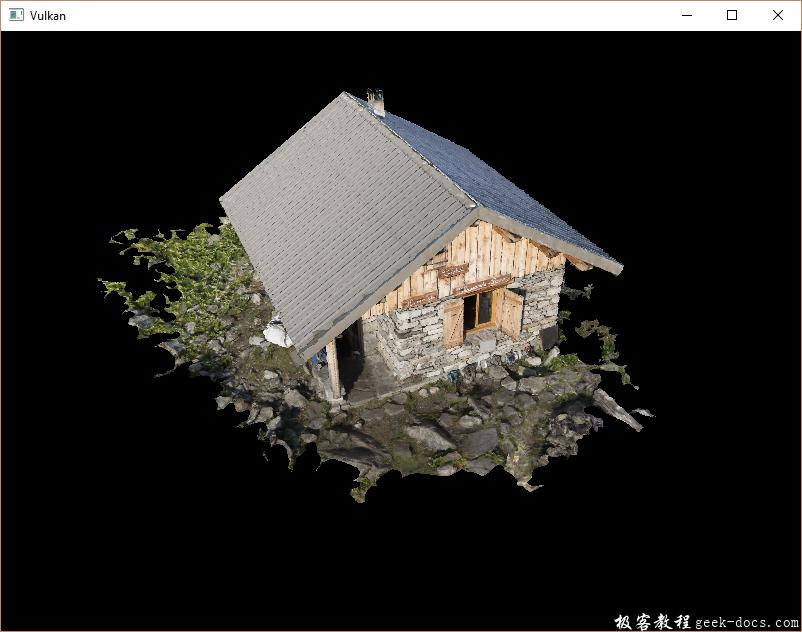# Vulkan 加载模型

## 准备工作

Library

Visual Studio## 样本模型

• chalet.obj
• chalet.jpg

const int WIDTH = 800;
const int HEIGHT = 600;

const std::string MODEL_PATH = "models/chalet.obj";
const std::string TEXTURE_PATH = "textures/chalet.jpg";


stbi_uc* pixels = stbi_load(TEXTURE_PATH.c_str(), &texWidth, &texHeight, &texChannels, STBI_rgb_alpha);


## 加载顶点和索引

std::vector<Vertex> vertices;
std::vector<uint32_t> indices;
VkBuffer vertexBuffer;
VkDeviceMemory vertexBufferMemory;


vkCmdBindIndexBuffer(commandBuffers[i], indexBuffer, 0, VK_INDEX_TYPE_UINT32);


#define TINYOBJLOADER_IMPLEMENTATION


void initVulkan() {
...
createVertexBuffer();
createIndexBuffer();
...
}

...

}


void loadModel() {
tinyobj::attrib_t attrib;
std::vector<tinyobj::shape_t> shapes;
std::vector<tinyobj::material_t> materials;
std::string err;

if (!tinyobj::LoadObj(&attrib, &shapes, &materials, &err, MODEL_PATH.c_str())) {
throw std::runtime_error(err);
}
}


OBJ文件由positions， normals， texture uvs 和 faces组成，其中每个顶点指向一个位置，通过索引指向 法线或者纹理坐标。这使得不仅可以重复使用整个顶点，还可以具有单独的属性。

attrib 容器持有所有的 positions， normals 和 texture uvs 在它的 attrib.vertices, attrib.normalsattrib.texcoords 容器中。 shapes 容器包含所有单独的对象和面。每个面由一组顶点组成，每个顶点包含 positions，normals 和 texture uvs 对应的 indices。OBJ模型也可以定义每个面的材质和纹理，但是我们忽略它们。

for (const auto& shape : shapes) {

}


for (const auto& shape : shapes) {
for (const auto& index : shape.mesh.indices) {
Vertex vertex = {};

vertices.push_back(vertex);
indices.push_back(indices.size());
}
}


vertex.pos = {
attrib.vertices[3 * index.vertex_index + 0],
attrib.vertices[3 * index.vertex_index + 1],
attrib.vertices[3 * index.vertex_index + 2]
};

vertex.texCoord = {
attrib.texcoords[2 * index.texcoord_index + 0],
attrib.texcoords[2 * index.texcoord_index + 1]
};

vertex.color = {1.0f, 1.0f, 1.0f};vertex.texCoord = {
attrib.texcoords[2 * index.texcoord_index + 0],
1.0f - attrib.texcoords[2 * index.texcoord_index + 1]
};## 删除顶点重复数据

#include <unordered_map>

...

std::unordered_map<Vertex, uint32_t> uniqueVertices = {};

for (const auto& shape : shapes) {
for (const auto& index : shape.mesh.indices) {
Vertex vertex = {};

...

if (uniqueVertices.count(vertex) == 0) {
uniqueVertices[vertex] = static_cast<uint32_t>(vertices.size());
vertices.push_back(vertex);
}

indices.push_back(uniqueVertices[vertex]);
}
}


bool operator==(const Vertex& other) const {
return pos == other.pos && color == other.color && texCoord == other.texCoord;
}


namespace std {
template<> struct hash<Vertex> {
size_t operator()(Vertex const& vertex) const {
return ((hash<glm::vec3>()(vertex.pos) ^
(hash<glm::vec3>()(vertex.color) << 1)) >> 1) ^
(hash<glm::vec2>()(vertex.texCoord) << 1);
}
};
}


#include <glm/gtx/hash.hpp>


## 结论

• Push constants
• Instanced rendering
• Dynamic uniforms
• Separate images and sampler descriptors
• Pipeline cache
• Multi subpasses

• 回顶